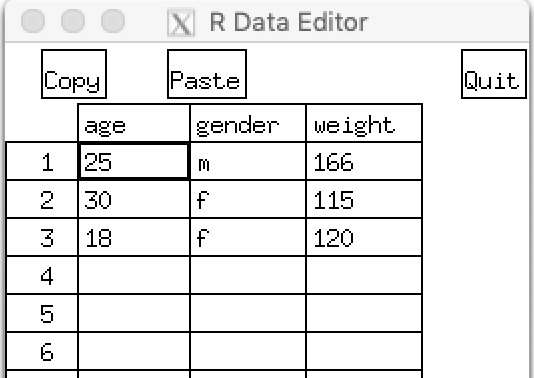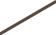# R语言教程：数据结构+导入数据！↑↑↑   关注选刊说   ↑↑↑

## 2.1 数据结构

R可以处理的数据类型(模式)包括数值型、字符型、逻辑型(TRUE/FALSE)、复数型(虚数)和原生型(字节)。
R拥有许多用于存储数据的对象类型，包括标量、向量、矩阵、数组、数据框和列表。

### 2. 向量

``a <- c(1, 2, 5, 3, 6, -2, 4) ``b <- c("one", "two", "three")``c <- c(TRUE, TRUE, TRUE, FALSE, TRUE, FALSE)``

``a <- c("k", "j", "h", "a", "c", "m")``a`` "h"``a[c(1, 3, 5)]`` "k" "h" "c"``a[2:6]        #冒号用于生成一个数值序列``  "j" "h" "a" "c" "m"``

### 3. 矩阵

``myymatrix <- matrix(vector, nrow=number_of_rows, ncol=number_of_columns, ``                    byrow=logical_value, dimnames=list(char_vector_rownames, char_vector_colnames))``
1. vector包含了矩阵的元素

2. nrow和ncol用以指定行和列的维数

3. 选项byrow则表明矩阵应当按行填充(byrow=TRUE)还是按列填充(byrow=FALSE)，默认情况下按列填充

4. dimnames包含了可选的、以字符型向量表示的行名和列名

``y <- matrix(1:20, nrow=5, ncol=4)   ``y``     [,1] [,2] [,3] [,4]    ``[1,]    1    6   11   16    ``[2,]    2    7   12   17    ``[3,]    3    8   13   18    ``[4,]    4    9   14   19    ``[5,]    5   10   15   20``

``cells <- c(1,26,24,68)``rnames <- c("R1", "R2")``cnames <- c("C1", "C2")``mymatrix <- matrix(cells, nrow=2, ncol=2, byrow=TRUE, ``                  dimnames=list(rnames, cnames))  ``mymatrix       ``   C1 C2    ``R1  1 26   ``R2 24 68``

``mymatrix <- matrix(cells, nrow=2, ncol=2, byrow=FALSE, ``                  dimnames=list(rnames, cnames))``mymatrix``   C1 C2``R1  1 24``R2 26 68``

``x <- matrix(1:10, nrow=2)  #创建了一个内容为数字1到10的2×5矩阵``x      ``     [,1] [,2] [,3] [,4] [,5]    ``[1,]    1    3    5    7    9    ``[2,]    2    4    6    8   10``x[2,]        #选择第二行的元素``  2  4  6  8 10 ``x[,2]        #选择第二列的元素`` 3 4 ``x[1,4]       #选择第1行的第4列的元素`` 7``x[1, c(4,5)] #选择第1行的第4和5列的元素`` 7 9``

### 4. 数组

``myarray <- array(vector, dimensions, dimnames)``
1. vector包含了数组中的数据

2. dimensions是一个数值型向量，给出各个维度下标的最大值

3. dimnames是可选的、各维度名称标签的列表

``dim1 <- c("A1", "A2")``dim2 <- c("B1", "B2", "B3")``dim3 <- c("C1", "C2", "C3", "C4")``z <- array(1:24, c(2, 3, 4), dimnames=list(dim1, dim2, dim3))  ``z    ``, , C1       ``   B1 B2 B3    ``A1  1  3  5    ``A2  2  4  6    ``, , C2       ``   B1 B2 B3    ``A1  7  9 11    ``A2  8 10 12    ``, , C3       ``   B1 B2 B3    ``A1 13 15 17    ``A2 14 16 18 ``   ``, , C4       ``   B1 B2 B3    ``A1 19 21 23    ``A2 20 22 24``

### 5. 数据框

``mydata <- data.frame(col1, col2, col3, ...)``
1. 列向量col1、col2、col3等可为任何类型(如字符型、数值型或逻辑型)

2. 每一列的名称可由函数names指定

``patientID <- c(1, 2, 3, 4)``age <- c(25, 34, 28, 52)``diabetes <- c("Type1", "Type2", "Type1", "Type1")``status <- c("Poor", "Improved", "Excellent", "Poor")``patientdata <- data.frame(patientID, age, diabetes, status)``patientdata      ``  patientID age diabetes    status    ``1         1  25    Type1      Poor    ``2         2  34    Type2  Improved    ``3         3  28    Type1 Excellent    ``4         4  52    Type1      Poor``

``patientdata[1:2]``  patientID age``1         1  25``2         2  34 ``3         3  28``4         4  52``patientdata[c("diabetes", "status")]``  diabetes    status``1    Type1      Poor    ``2    Type2  Improved    ``3    Type1 Excellent    ``4    Type1      Poor``patientdata\$age         # \$用来选取一个给定数据框中的某个特定变量`` 25 34 28 52``

#### attach()、detach()和with()

``summary(mtcars\$mpg)``plot(mtcars\$mpg, mtcars\$disp)``plot(mtcars\$mpg, mtcars\$wt)``

``attach(mtcars)    #函数attach()可将数据框添加到R的搜索路径中``summary(mpg)``plot(mpg, disp)``plot(mpg, wt)``detach(mtcars)    #函数detach()将数据框从搜索路径中移除``

detach()并不会对数据框本身做任何处理，可以省略，但其实它应当被例行地放入代码中。

``mpg <- c(25, 36, 47)``attach(mtcars)``The following object(s) are masked _by_ '.GlobalEnv':     mpg``plot(mpg, wt)``Error in xy.coords(x, y, xlabel, ylabel, log) :``      'x' and 'y' lengths differ``mpg    `` 25 36 47``

``with(mtcars, {``  print(summary(mpg))``  plot(mpg, disp)``  plot(mpg, wt)``})``

``with(mtcars, {``  stats <- summary(mpg)``  stats``})``stats``错误: 找不到对象'stats'``

``with(mtcars, {``  nokeepstats <- summary(mpg)``  keepstats <<- summary(mpg)``})``nokeepstats   ``错误: 找不到对象'nokeepstats'``keepstats`` Min. 1st Qu.  Median    Mean 3rd Qu.    Max.       ``10.40   15.43   19.20   20.09   22.80   33.90``

#### 实例标识符

``patientdata<-data.frame(patientID, age, diabetes, ``                        status, row.names=patientID)`    `

### 6. 因子

• 名义型变量是没有顺序之分的类别变量

• 有序型变量表示一种顺序关系，而非数量关系

• 连续型变量可以呈现为某个范围内的任意值，并同时表示了顺序和数量
类别(名义型)变量和有序类别(有序型)变量在R中称为
因子(factor)

#### 类别(名义型)变量

``diabetes <- c("Type1", "Type2", "Type1", "Type1")``diabetes <- factor(diabetes)``

#### 有序型变量

``status <- c("Poor", "Improved", "Excellent", "Poor")``status <- factor(status, ordered=TRUE)``

``status <- factor(status, order=TRUE,``                 levels=c("Poor", "Improved", "Excellent"))``

#### 数值型变量

``sex <- factor(sex, levels=c(1, 2), labels=c("Male", "Female"))``

``#以向量形式输入数据``patientID <- c(1, 2, 3, 4)``age <- c(25, 34, 28, 52)``diabetes <- c("Type1", "Type2", "Type1", "Type1")``status <- c("Poor", "Improved", "Excellent", "Poor")``#指定为一个普通因子``diabetes <- factor(diabetes)   ``#指定为一个有序型因子``status <- factor(status, order=TRUE)         ``patientdata <- data.frame(patientID, age, diabetes, status``#显示对象的结构``str(patientdata)``'data.frame':  4 obs. of  4 variables:     ``\$ patientID: num  1 2 3 4     ``\$ age      : num  25 34 28 52     ``\$ diabetes : Factor w/ 2 levels "Type1","Type2": 1 2 1 1                 ``\$ status   : Ord.factor w/ 3 levels "Excellent"<"Improved"<..: 3 2 1 3  ``

diabetes是一个因子；status是一个有序型因子。

``#显示对象的统计概要``summary(patientdata)  `` patientID         age         diabetes       status       ``Min.   :1.00   Min.   :25.00   Type1:3   Excellent:1       ``1st Qu.:1.75   1st Qu.:27.25   Type2:1   Improved :1       ``Median :2.50   Median :31.00             Poor     :2       ``Mean   :2.50   Mean   :34.75                               ``3rd Qu.:3.25   3rd Qu.:38.50                               ``Max.   :4.00   Max.   :52.00``

### 7. 列表

``mylist <- list(object1, object2, ...)``

``mylist <- list(name1=object1, name2=object2, ...)``

``g <- "My First List"``h <- c(25, 26, 18, 39)``j <- matrix(1:10, nrow=5)``k <- c("one", "two", "three")``mylist <- list(title=g, ages=h, j, k)      #创建列表``mylist                                     #输出整个列表``\$title     "My First List"``\$ages    `` 25 26 18 39    ``[]         ``      [,1] [,2]    ``[1,]    1    6    ``[2,]    2    7    ``[3,]    3    8    ``[4,]    4    9    ``[5,]    5   10    ``[]    `` "one"   "two"   "three"``mylist[]                                #输出第二个成分`` 25 26 18 39``mylist[["ages"]]`` 25 26 18 39``

#### 注意事项

• 对象名称中的句点(.)没有特殊意义
R中用美元符号(\$)指定一个数据框或列表中的某些部分。

• 将一个值赋给某个向量、矩阵、数组或列表中一个不存在的元素时，R将自动扩展这个数据结构以容纳新值。

``x <- c(8, 6, 4)``x <- 10``x            #通过赋值，向量x由三个元素扩展到了七个元素``  8  6  4 NA NA NA 10``x <- x[1:3]  #重新将其缩减回三个元素``x    `` 8 6 4``
• R中没有标量。标量以单元素向量的形式出现。

• R中的下标不从0开始，而从1开始。在上述向量中，x的值为8。

• 变量无法被声明。它们在首次被赋值时生成。

## 2.2 数据输入

### 1. 键盘输入数据

#### 文本编辑器

edit()会自动调用一个允许手动输入数据的文本编辑器。

``mydata <- data.frame(age=numeric(0), gender=character(0), weight=numeric(0))``edit(mydata)         #通过图2-1所示文本编辑器输入数据，但结果并未保存``  age gender weight    ``1  25      m    166    ``2  30      f    115    ``3  18      f    120    ``Warning message:    ``In edit.data.frame(mydata) : 在'gender'里加上了因子水准``图2-1 通过文本编辑器输入数据

``mydata <- edit(mydata)  #编辑的结果需要赋值回对象本身，结果保存在mydata中``mydata``  age gender weight    ``1  25      m    166    ``2  30      f    115    ``3  18      f    120``

#### 直接嵌入数据集

``mydatatxt <- "`` age gender weight`` 25 m 166`` 30 f 115`` 18 f 120`` "``mydata <- read.table(header=TRUE, text=mydatatxt)   ``#read.table()函数被用于处理字符串并返回数据框``

### 2. 从带分隔符的文本文件导入数据

`mydataframe<-read.table(file,options)`

1. file是一个带分隔符的ASCII文本文件

2. options是控制如何处理数据的选项

 选项 描述 header 一个表示文件是否在第一行包含了变量名的逻辑型变量 sep 分开数据值的分隔符 默认是 sep=""，这表示了一个或多个空格、制表符、换行或回车 sep=","读取用逗号来分隔行内数据的文件  sep="\t"读取使用制表符来分割行内数据的文件 row.names 一个用于指定一个或多个行标记符的可选参数 col.names 如果数据文件的第一行不包括变量名(header=FASLE)，可以用 col.names 去指定一个包含变量名的字符向量 colClasses 可以对每一列都指定一个类(比如说，逻辑型、数值型、字符型或因子型)

StudentID,First,Last,Math,Science,Social Studies
011,Bob,Smith,90,80,67
012,Jane,Weary,75,,80
010,Dan,"Thornton, III",65,75,70
040,Mary,"O'Leary",90,95,92

``setwd("~/目标文件所在文件夹")         #定位到目标文件所在文件夹``grades <‐ read.table("studentgrades.csv", header=TRUE,``    row.names="StudentID", sep=",")  ``grades                             #显示导入数据``   First          Last Math Science Social.Studies    ``11   Bob         Smith   90      80             67    ``12  Jane         Weary   75      NA             80    ``10   Dan Thornton, III   65      75             70    ``40  Mary       O'Leary   90      95             92``str(grades)    ``'data.frame':  4 obs. of  5 variables:     ``\$ First         : Factor w/ 4 levels "Bob","Dan","Jane",..: 1 3 2 4     ``\$ Last          : Factor w/ 4 levels "O'Leary","Smith",..: 2 4 3 1     ``\$ Math          : int  90 75 65 90     ``\$ Science       : int  80 NA 75 95     ``\$ Social.Studies: int  67 80 70 92``

1. 列StudentID现在是行名，不再有标签，也失去了前置的0

2. Dan的姓左右使用引号，避免Thornton和III之间的空格

3. O’Leary左右使用引号，否则R会把单引号读取为分隔符

5. 可以用colClasses 选项去对每一列都指定一个类

``grades <- read.table("studentgrades.csv", header=TRUE,``    row.names="StudentID", sep=",",``    colClasses=c("character", "character", "character",``                 "numeric", "numeric", "numeric"))``grades        ``    First          Last Math Science Social.Studies    ``011   Bob         Smith   90      80             67    ``012  Jane         Weary   75      NA             80    ``010   Dan Thornton, III   65      75             70    ``040  Mary       O'Leary   90      95             92``str(grades)    ``'data.frame':  4 obs. of  5 variables:     ``\$ First         : chr  "Bob" "Jane" "Dan" "Mary"     ``\$ Last          : chr  "Smith" "Weary" "Thornton, III" "O'Leary"     ``\$ Math          : num  90 75 65 90     ``\$ Science       : num  80 NA 75 95     ``\$ Social.Studies: num  67 80 70 92``

### 3. 导入Excel数据

#### 可以用xlsx包直接导入

`read.xlsx(file,n)`

1. file是Excel工作簿的所在路径
2. n则为要导入的工作表序号

``library(xlsx)``workbook <- "目标文件夹/studentgrades.xlsx"``mydataframe <- read.xlsx(workbook, 1)``

R还可以从流行的统计软件、特殊格式的文件、多种关系型数据库管理系统、专业数据库、网站和在线服务中导入数据。具体方法可参见原书。

## 2.3 数据集的标注

### 1. 变量标签——为变量名添加描述性的标签

``names(patientdata) <- "Age at hospitalization (in years)"``patientdata``  patientID Age at hospitalization (in years) diabetes    status    ``1         1                                25    Type1      Poor    ``2         2                                34    Type2  Improved    ``3         3                                28    Type1 Excellent    ``4         4                                52    Type1      Poor``

``patientdata      ``  Age at hospitalization (in years)    ``1                                25    ``2                                34    ``3                                28    ``4                                52``

### 2. 值标签——为类别型变量中的编码添加值标签

``patientdata\$gender <- factor(patientdata\$gender,``                           levels = c(1,2),``                           labels = c("male", "female"))``
1. levels代表变量的实际值

2. labels表示包含了理想值标签的字符型向量

## 2.4 处理数据对象的实用函数

 函数 用途 length(object) 显示对象中元素/成分的数量 dim(object) 显示某个对象的维度 str(object) 显示某个对象的结构 class(object) 显示某个对象的类或类型 mode(object) 显示某个对象的模式 names(object) 显示某对象中各成分的名称 c(object, object,...) 将对象合并入一个向量 cbind(object, object, ...) 按列合并对象 rbind(object, object, ...) 按行合并对象 object 输出某个对象 head(object) 列出某个对象的开始部分 tail(object) 列出某个对象的最后部分 ls() 显示当前的对象列表 rm(object, object, ...) 删除一个或更多个对象语句 rm(list = ls())将删除当前工作环境中的几乎所有对象 newobject<- edit(object) 编辑对象并另存为 newobject fix(object) 直接编辑对象## 手把手教你用Excel轻松绘制-簇状柱形图

2020-12-30 18:54:34

## 90后科研女博士出名了！她把这种鲜花当面膜敷，皮肤白嫩得让男人受不了！

2020-12-30 19:25:14